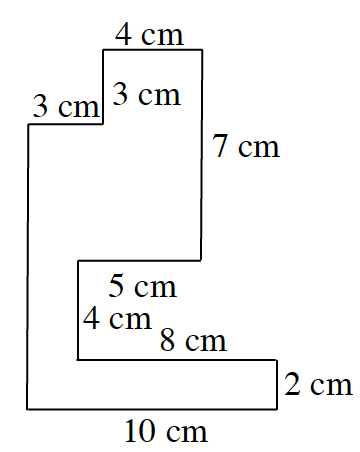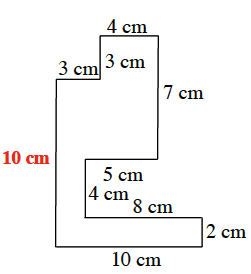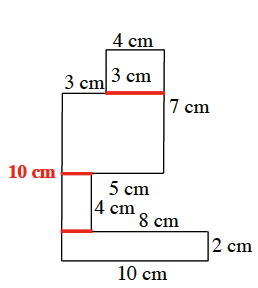### Home > ACC6 > Chapter 8 Unit 8 > Lesson CC1: 8.2.1 > Problem8-81

8-81.

Find the area and perimeter of the figure shown below. All angles are right angles. Show your work clearly.Find the missing side. Unknown side length for the figure is added, 10 centimeters.

Add up all the sides to find the perimeter.

The perimeter is $56$ cm.

Break up the figure into rectangles.

Find the area of each rectangle and add them together to find the area of the figure.

$20 \text{ sq cm} + 8 \text{ sq cm} + 28 \text{ sq cm} + \\ 12 \text{ sq cm} = 68 \text{ sq cm}$